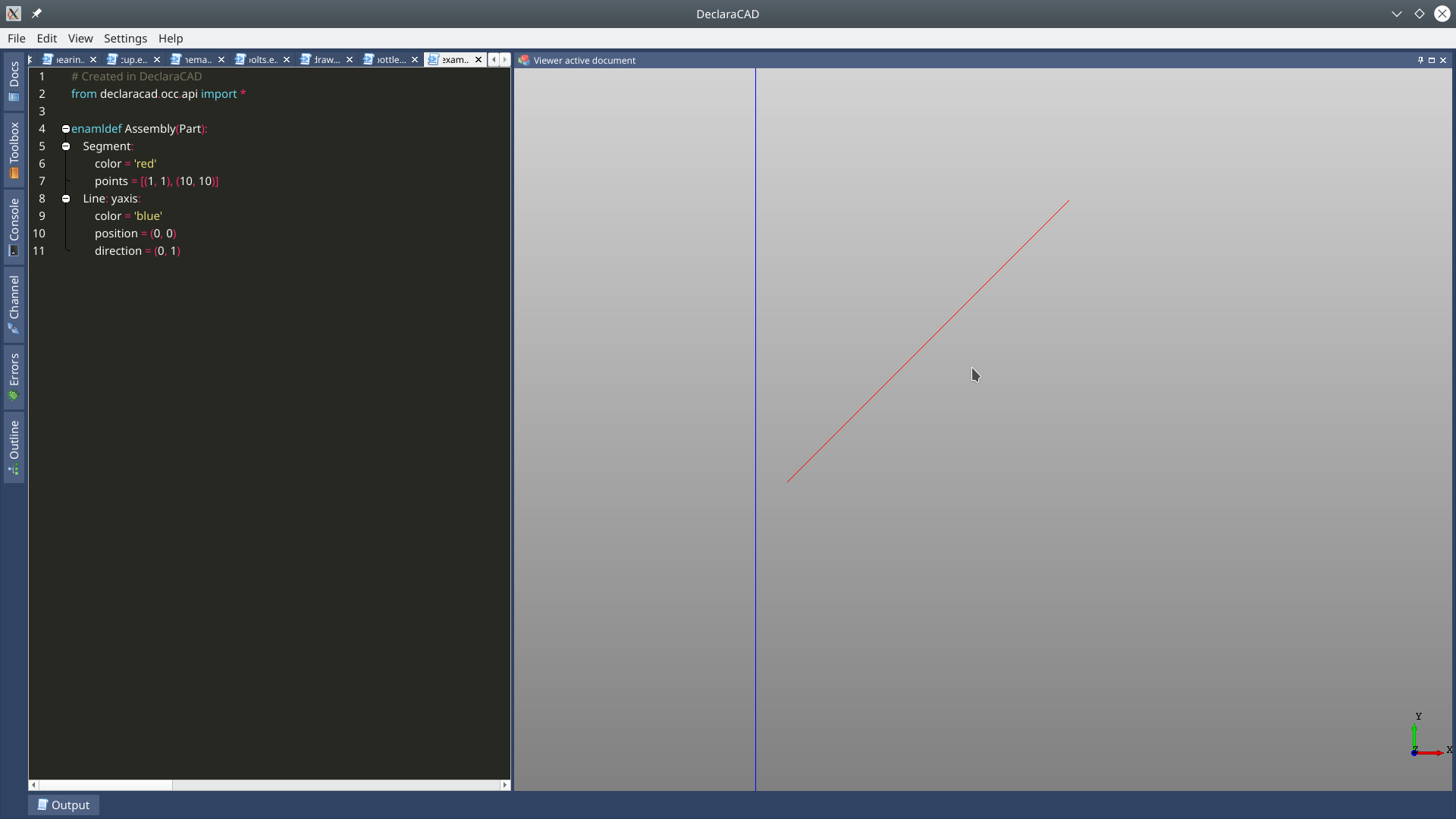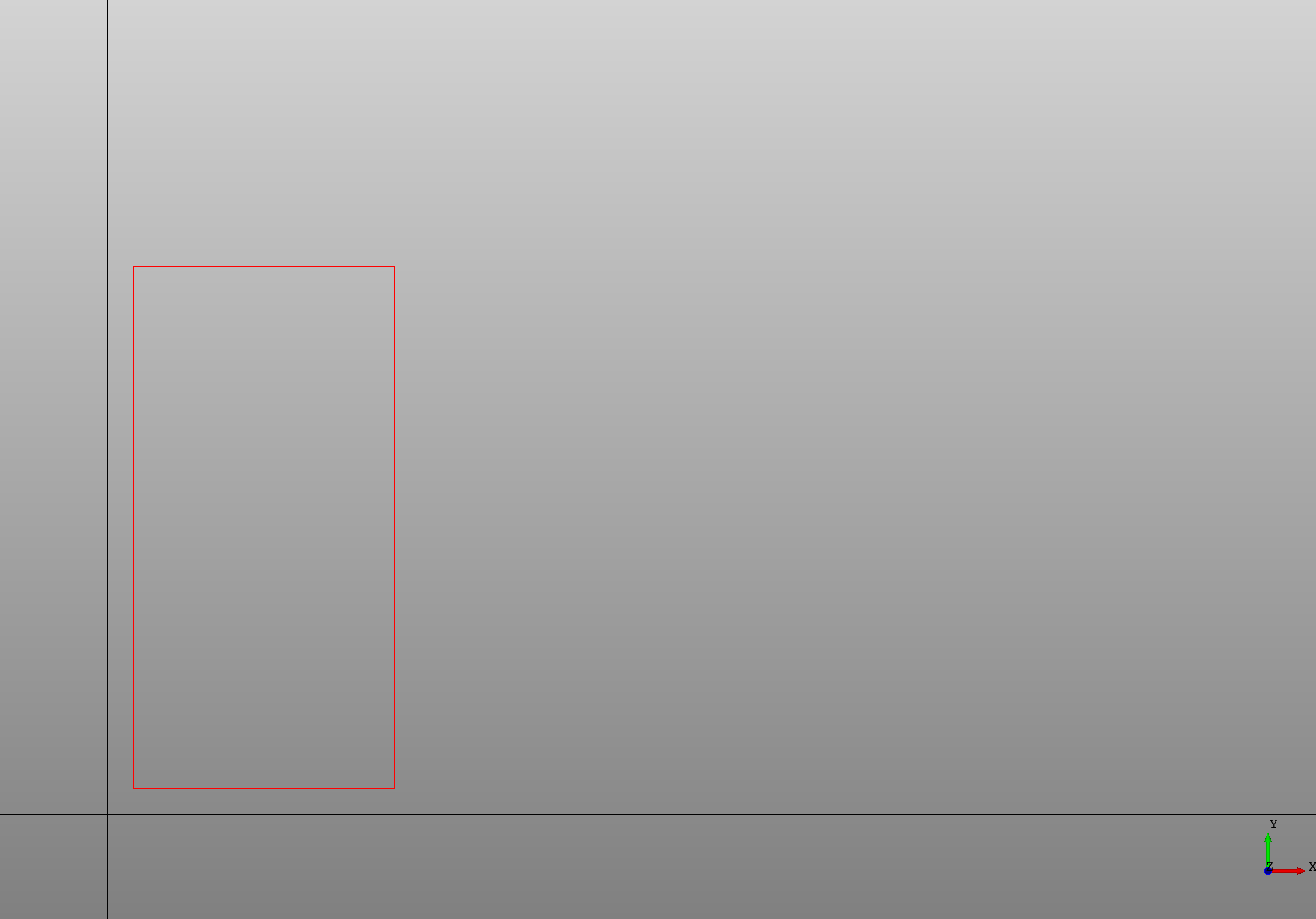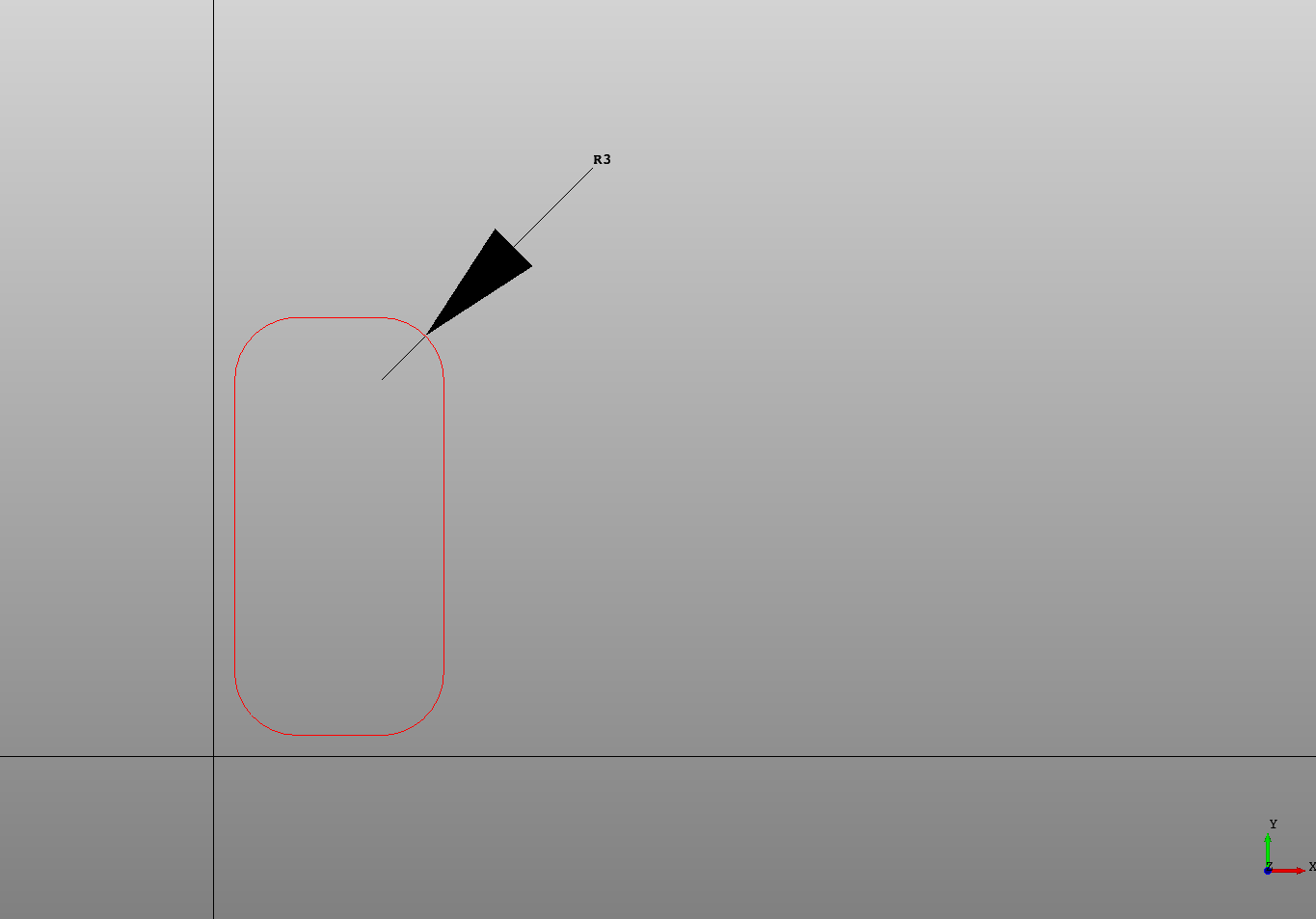## Lines and Segments

A straight line can be created in DeclaraCAD using the `Line` or `Segment` declarations.

A `Line` and `Segment` can both be specified using the `points` attribute with two points.

A `Line` may be also defined with only a `position` and `direction`.

``````# Created in DeclaraCAD

enamldef Assembly(Part):
Segment:
color = 'red'
points = [(1, 1), (10, 10)]
Line: yaxis:
color = 'blue'
position = (0, 0)
direction = (0, 1)
``````The difference between the two is the `Line` is unbounded / of infinite length while a `Segment` is just between a start and end points.

Typically the `Segment` will be used for sketching as it creates an edge that can be converted into a `Wire`, while a `Line` can be used to determine intersections.

## Rectangles

DeclaraCAD includes a `Rectangle` declaration that builds a Wire from a set of line segments. The rectangle's origin is at the bottom left corner of the xy plane and is defined by the `width` and `height`.

Note: The `Rectangle` shape will return a wire by default, use `as_face = True` to convert the result into a face.

``````# Created in DeclaraCAD

enamldef Assembly(Part):
Rectangle:
color = 'red'
position = (1, 1)
width = 10
height = 20

# For reference
Line: xaxis:
color = 'black'
direction = (1, 0)
Line: yaxis:
color = 'black'
direction = (0, 1)
``````You can also specify radius's for the corners. Using `rx` and `ry`. If only one is given it will use the same for both.

``````# Created in DeclaraCADTo orient the wire in another plane use the `direction` attribute or wrap it in a `Transform`.